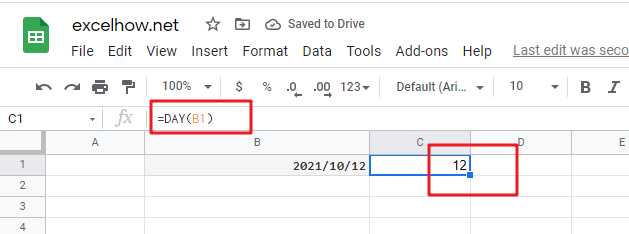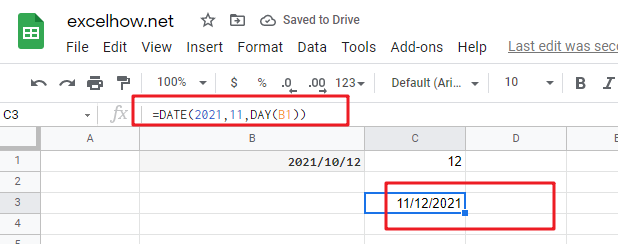# ExcelHow

This post will guide you how to use Google Sheets DAY function with syntax and examples.

## Description

The Google Sheets DAY returns a day of a date (from 1 to 31). You can use the DAY function to extract a day number from a given date in google sheets.

The DAY function can be used to get the day of the month as number between 1 to 31 from a given date. The purpose of this function is to get the day as number from a date and its returned values is a number indicating the day component in a date.

The DAY function is a build-in function in Google Sheets and it is categorized as a Date function.

## Syntax

The syntax of the DAY function is as below:

=DAY(date_value)

Where the DAY function arguments is:

• Date_text -This is a required argument. The date from which to extract the day. it can be a cell reference containing a date or a function returning a date type or a number.

Note:

• The date_value argument must be a valid date value, or it will return the #VALUE! Error.

## Google Sheets DAY Function Examples

The below examples will show you how to use google sheets DAY Function to return the day of a date.

#1 To extract the day of the month from a given date value in Cell B1, just using the following DAY function:

`=DAY(B1)`Note:  The above excel formula will return the day of the date in Cell B1.

#2 the DAY function can be used to extract a day value and then passed the day value into another function called DATE function. Like below:

`=DATE(2021,11,DAY(B1))`